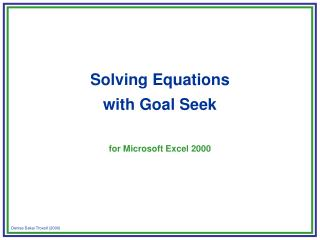# Solving Equations with Goal Seek for Microsoft Excel 2000 - PowerPoint PPT PresentationDownload PresentationSolving Equations with Goal Seek for Microsoft Excel 2000

Solving Equations with Goal Seek for Microsoft Excel 2000
Download Presentation## Solving Equations with Goal Seek for Microsoft Excel 2000

- - - - - - - - - - - - - - - - - - - - - - - - - - - E N D - - - - - - - - - - - - - - - - - - - - - - - - - - -
##### Presentation Transcript

1. Solving Equations with Goal Seek for Microsoft Excel 2000

2. A Simple Problem • grades, grades, grades*… • You would love to have a quiz average of 80 points (or better) in the QTM1300 course by the end of the semester. • Your quiz scores on the first nine quizzes are given in the table below. • What score do you need to earn on the 10th and final quiz in order to meet your goal average of 80 points? * from FCL Excel Notes by Joyce Gordon, Babson College, 1998.

3. Quiz Average 90 + 88 + 78 + 68 + 84 + 82 + 66 + 74 + 72 + x = 80 10 Formulate the Problem • obtain equation to be solved You are looking for x = score on 10th quiz so that NOTE: Only use Goal Seek if the equation • contains only one variable; • has the right-hand side equal to a constant.

4. Preparing the Worksheet for Goal Seek • start with a blank sheet

5. Preparing the Worksheet for Goal Seek • enter labels and constants Enter labels in cells A1:A13 and B1

6. Preparing the Worksheet for Goal Seek • enter labels and constants NOTE: These labels are not essential for the use of Goal Seek

7. Remember… • Quiz • 1 • 2 • 3 • 4 • 5 • 6 • 7 • 8 • 9 • Score • 90 • 88 • 78 • 68 • 84 • 82 • 66 • 74 • 72 Preparing the Worksheet for Goal Seek • enter labels and constants Enter constants in cells B2:B10

8. Remember… 90 + 88 + 78 + 68 + 84 + 82 + 66 + 74 + 72 + x = 80 10 x Preparing the Worksheet for Goal Seek • enter formula Variable values in cell B11 Left-hand side of equation in cell B13 NOTE: These cells will be colored to indicate that they are essential for Goal Seek

9. Remember… 90 + 88 + 78 + 68 + 84 + 82 + 66 + 74 + 72 + x = 80 10 Preparing the Worksheet for Goal Seek • enter formula Click on cell B13

10. Remember… 90 + 88 + 78 + 68 + 84 + 82 + 66 + 74 + 72 + x = 80 10 Preparing the Worksheet for Goal Seek • enter formula Type in =AVERAGE(

11. Remember… 90 + 88 + 78 + 68 + 84 + 82 + 66 + 74 + 72 + x = 80 10 Preparing the Worksheet for Goal Seek • enter formula Highlight cells B2:B11

12. Remember… 90 + 88 + 78 + 68 + 84 + 82 + 66 + 74 + 72 + x = 80 10 Preparing the Worksheet for Goal Seek • enter formula Type in ) and hit Enter

13. Preparing the Worksheet for Goal Seek • enter formula NOTE: Alternative formula for cell B13: =(B2+ B3+ B4+ B5+ B6+ B7+ B8+ B9+ B10+ B11)/10

14. Preparing the Worksheet for Goal Seek • enter formula NOTE: The function AVERAGE entered in cell B13 returns the average of the values in cells B2:B11; blank cells are ignored

15. Using Goal Seek • invoke Goal Seek Click on Tools

16. Using Goal Seek • invoke Goal Seek Click on Goal Seek

17. Using Goal Seek • invoke Goal Seek

18. NOTE: The cell displayed in the Set cell: box must contain the formula for the left-hand side of the equation (cell B13) Using Goal Seek • complete the Goal Seek dialog box Click on cell B13

19. Using Goal Seek • complete the Goal Seek dialog box Click on the To value: box

20. Using Goal Seek • complete the Goal Seek dialog box Type in 80 NOTE: The value displayed in the To value: box must be the constant on the right-hand side of the equation (80)

21. Using Goal Seek • complete the Goal Seek dialog box Click on the By changing cell: box

22. Using Goal Seek • complete the Goal Seek dialog box Click on cell B11 NOTE: The cell displayed in the By changing cell: box must be the cell containing variable values (cell B11)

23. Using Goal Seek • execute Goal Seek Click on OK

24. Using Goal Seek • read solution You need to earn a score of 98 on the 10th quiz to meet your goal average of 80 points. NOTE: If the equation has multiple solutions, Goal Seek usually finds the closest solution to the initial variable value.

25. Back to studying! Using Goal Seek • end execution Click on OK

26. Final Comments • Goal Seek might not find the exact solution… • Goal Seek has a precision of 0.001: • when the variable value returned by Goal Seek is plugged into the left-hand side of the equation, the result will be within 0.001 of the right-hand side; • use Excel Solver for higher precision and/or equations with multiple variables.